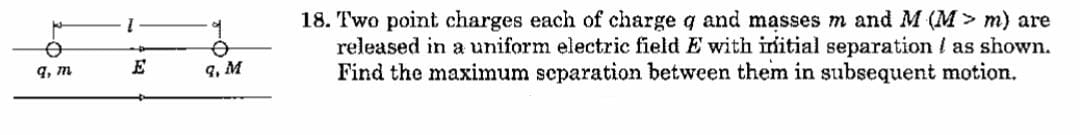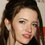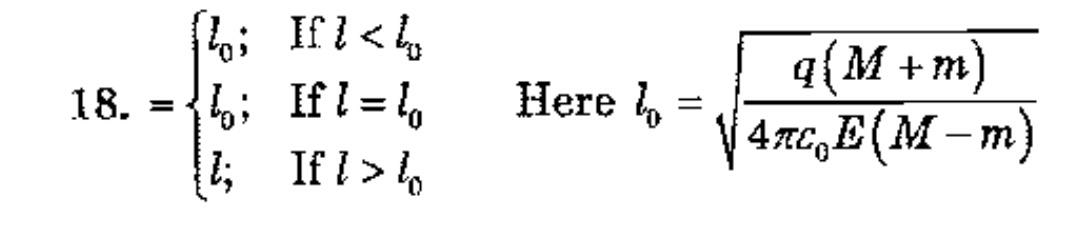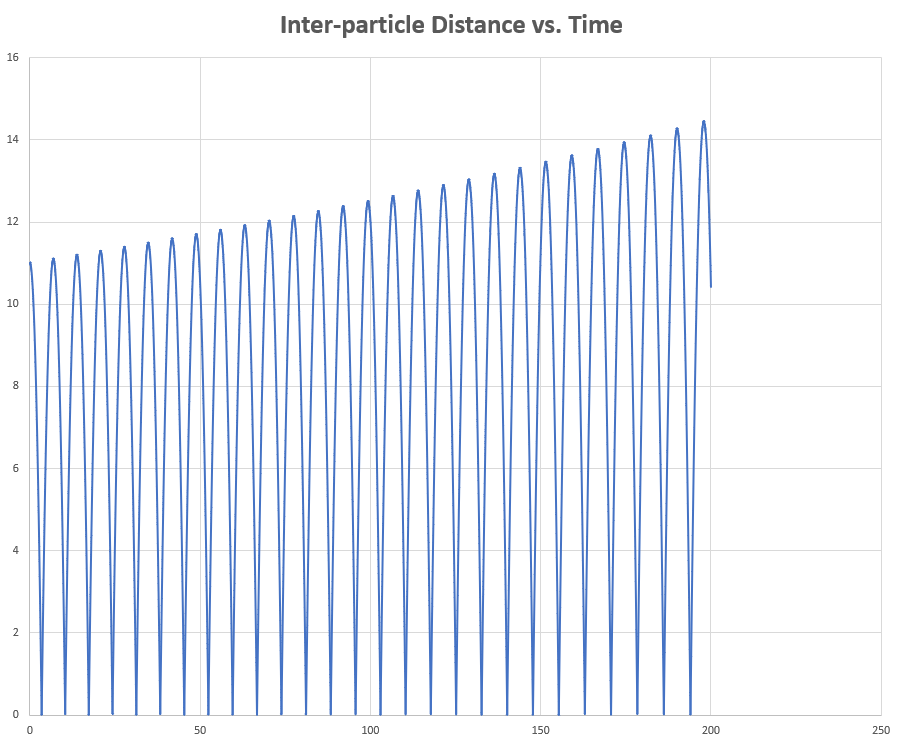# Electric field and force

Can we solve this problem AnaylticallyI will share my attempt within some hours.Note by Talulah Riley
7 months, 1 week ago

This discussion board is a place to discuss our Daily Challenges and the math and science related to those challenges. Explanations are more than just a solution — they should explain the steps and thinking strategies that you used to obtain the solution. Comments should further the discussion of math and science.

When posting on Brilliant:

• Use the emojis to react to an explanation, whether you're congratulating a job well done , or just really confused .
• Ask specific questions about the challenge or the steps in somebody's explanation. Well-posed questions can add a lot to the discussion, but posting "I don't understand!" doesn't help anyone.
• Try to contribute something new to the discussion, whether it is an extension, generalization or other idea related to the challenge.
• Stay on topic — we're all here to learn more about math and science, not to hear about your favorite get-rich-quick scheme or current world events.

MarkdownAppears as
*italics* or _italics_ italics
**bold** or __bold__ bold
- bulleted- list
• bulleted
• list
1. numbered2. list
1. numbered
2. list
Note: you must add a full line of space before and after lists for them to show up correctly
paragraph 1paragraph 2

paragraph 1

paragraph 2

[example link](https://brilliant.org)example link
> This is a quote
This is a quote
    # I indented these lines
# 4 spaces, and now they show
# up as a code block.

print "hello world"
# I indented these lines
# 4 spaces, and now they show
# up as a code block.

print "hello world"
MathAppears as
Remember to wrap math in $$ ... $$ or $ ... $ to ensure proper formatting.
2 \times 3 $2 \times 3$
2^{34} $2^{34}$
a_{i-1} $a_{i-1}$
\frac{2}{3} $\frac{2}{3}$
\sqrt{2} $\sqrt{2}$
\sum_{i=1}^3 $\sum_{i=1}^3$
\sin \theta $\sin \theta$
\boxed{123} $\boxed{123}$

## Comments

Sort by:

Top Newest

Is the answer:

$d_{max} = \frac{q(M+m)}{4 \pi \epsilon_o EL(M-m)}$

- 7 months, 1 week ago

Log in to reply

@Karan Chatrath No,but very very near to correct answer. I am not telling you answer because you always say, don't tell answer.

- 7 months, 1 week ago

Log in to reply

Maybe I am missing something. Cannot spot my mistake.

- 7 months, 1 week ago

Log in to reply

@Karan Chatrath if you don't mind me, your answer is also incorrect with dimension.
By the way, do you like to see answer?

- 7 months, 1 week ago

Log in to reply

I think my answer is dimensionally correct. Please recheck this. Also, you may share the correct answer.

- 7 months, 1 week ago

Log in to reply

@Karan Chatrath OMG there is a coincidence if you watch answer basically the $L$ in your answer is managing the need of square root.That's really sexy.

- 7 months, 1 week ago

Log in to reply

I think that the book is wrong here. I have done a numerical simulation and checked the expression that I got and the one in the book, and the one I have obtained seems correct. I even checked various possible cases by varying the initial separation parameter.

@Steven Chase could you validate this observation when possible?

- 7 months, 1 week ago

Log in to reply

I'm not even convinced that there is a maximum distance. I got the following results with the numerical values given in the code. It looks like the local maxima might just keep increasing.1 2 3 4 5 6 7 8 9 10 11 12 13 14 15 16 17 18 19 20 21 22 23 24 25 26 27 28 29 30 31 32 33 34 35 36 37 38 39 40 41 42 43 44 45 46 47 48 49 50 51 52 import math dt = 10.0**(-5.0) q = 2.0 m = 3.0 M = 5.0 E = 7.0 L = 11.0 e0 = 1.0 k = 1.0/(4.0*math.pi*e0) Dref = q*(M+m)/(4.0*math.pi*e0*E*L*(M-m)) ################################# t = 0.0 count = 0 xm = 0.0 xM = L xmd = 0.0 xMd = 0.0 xmdd = 0.0 xMdd = 0.0 while t <= 200.0: xm = xm + xmd*dt xM = xM + xMd*dt xmd = xmd + xmdd*dt xMd = xMd + xMdd*dt D = xM - xm F = k*q*q/(D**2.0) Fm = q*E - F FM = q*E + F xmdd = Fm/m xMdd = FM/M t = t + dt count = count + 1 if count % 1000 == 0: print t,D 

- 7 months, 1 week ago

Log in to reply

I ran a quick simulation with your code and I see that the progressively increasing separation is a numerical issue that you seem to be having. If I use the explicit Euler solver, I get a result similar to yours. I am using a higher-order accurate integrator.

- 7 months, 1 week ago

Log in to reply

Yes, I neglected to check with multiple time steps and compare. When I run with a microsecond time step, the drift upwards is much smaller, but still there. It almost seems as if with a very small time step, the particle separation will oscillate between $L$ and zero in perpetuity.

- 7 months, 1 week ago

Log in to reply

I think it oscillates between $0.083$ and $L$. This is the value I get with the expression and also validated using simulations. From the plots, it looks like zero as 0.083 is a relatively small magnitude.

- 7 months ago

Log in to reply

So the max separation is $L$ for those numbers. But the expression doesn't reduce to $L$, does it?

- 7 months ago

Log in to reply

Indeed it does not. For the numbers you have chosen, the expression yields the minimum separation

- 7 months ago

Log in to reply

I'm getting the impression that the qualitative behavior depends on the particular constants chosen.

- 7 months ago

Log in to reply

Yes, initially, I just solved for the fixed points of $\dot{x}=f(x)$ and thought that my job is done. But when @Lil Doug shared the answer with me, showing the various cases, I realised that I had not thought it through. Having said that, I do think that the expression that the book shows is not correct.

- 7 months ago

Log in to reply

I ran the simulation using the following parameters and got the result with matches with the expression that I derived. While testing with closed-form expressions, I like to use irrational numbers as parameters.

$q = \sqrt{2}$ $E = \sqrt{5}$ $L = \frac{\pi}{20}$ $m = \mathrm{e}$ $M = \mathrm{e}^2$ $\epsilon_o = 1$

- 7 months, 1 week ago

Log in to reply

I look forward to seeing your attempt, after which I will share mine. Later, though.

- 7 months, 1 week ago

Log in to reply

@Steven Chase My attempt:

Coordinate of $M$ is $x_1$ and that of $m$ is $x_2$.

Let:

$x = x_1 - x_2$

Applying Newton's second law gives:

$M\ddot{x}_1 = qE + \frac{Kq^2}{x^2}$ $m\ddot{x}_2 = qE - \frac{Kq^2}{x^2}$

Manipulating and rearranging both equations gives:

$\ddot{x}_1 - \ddot{x}_2 = \frac{Kq^2}{x^2}\left(\frac{1}{M} + \frac{1}{m}\right) + qE\left(\frac{1}{M} - \frac{1}{m}\right)$

$\implies \ddot{x} = A + \frac{B}{x^2}$

$A = qE\left(\frac{1}{M} - \frac{1}{m}\right)$ $B =Kq^2\left(\frac{1}{M} + \frac{1}{m}\right)$

$x(0) = L$ $\dot{x}(0) = 0$

$\ddot{x} = A + \frac{B}{x^2}$ $\implies \dot{x} d(\dot{x}) = \left(A + \frac{B}{x^2}\right) \ dx$

Integrating gives:

$\frac{\dot{x}^2}{2} = A + \frac{B}{x^2} + C$

Apply initial conditions and solve for integration constant $C$. Finally, the separation is max or min when $\dot{x} = 0$. Equating $\dot{x}=0$ gives a quadratic equations, one root of which is obviously $L$. The other is:

$d = \frac{q(M+m)}{4 \pi \epsilon_o EL(M-m)}$

Whether $d$ is a max or min can be confirmed by a second derivative test, which gives rise to cases.

- 7 months, 1 week ago

Log in to reply

Does this method distinguish between local and global maxima?

- 7 months, 1 week ago

Log in to reply

Since we are solving a quadratic equation which has only two solutions, the local maxima and minima are actually the global maxima and minima.

- 7 months, 1 week ago

Log in to reply

@Karan Chatrath in the 3rd line of your solution it will be $m$

- 7 months ago

Log in to reply

Nice catch. Corrected now.

- 7 months ago

Log in to reply

@Lil Doug Bro your problems are really hard. Lol.

- 7 months ago

Log in to reply

×

Problem Loading...

Note Loading...

Set Loading...